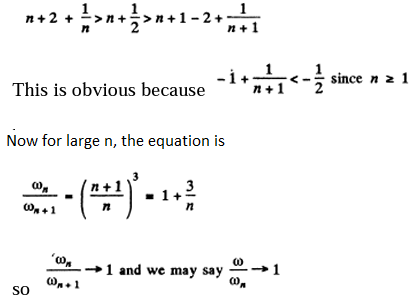# I.E. Irodov Solutions on Scattering of Particles Rutherford-Bohr Atom

Scattering of Particles Rutherford-Bohr Atom is a chapter that mainly deals with the scattering of particles. The students of Class 12 must pay attention to this topic as it plays a key role in understanding the basic concepts of atoms and other particles. IE IRODOV Physics Solutions provide solutions to various problems related to atoms and molecules. This helps in better understanding and grip over the subject.

The in-depth explanation of problems with related equations and diagrams aids the students to grasp easily and the concepts are engraved better in their minds. The problems and solutions provided here help the student to clear the competitive exams easily as the solutions go in flow with the theoretical points and relate with the practical applications. The numerical are solved in a complete manner with appropriate formulae, equations, diagrams and units. A diverse variety of questions are solved to help the students to practise and score higher marks in their upcoming exams.

### I.E. Irodov Solutions on Scattering of Particles Rutherford-Bohr Atom

1. Employing Thomson's model, calculate the radius of a hydrogen atom and the wavelength of emitted light if the ionization energy of the atom is known to be equal to E = 13.6 eV.

Solution:

1. The Thomson model consists of a uniformly charged nucleus in which the electrons are at rest at certain equilibrium points (the plum in the pudding model). For the hydrogen nucleus, the charge on the nucleus is + e while the change on the electron is electron is -e. The electron by symmetry must be at the centre of the nuclear charge where the potential is

$\phi _{0}=(\frac{1}{4\pi \varepsilon _{o}})(\frac{3e}{2R})$

where R is the radius of the nuclear charge distribution. The potential energy of the electron is -eϕo and since the electron is at rest, and this is also the total energy. To ionise such an energetic electron will require an energy of E=eϕo

From this we find

$R=(\frac{1}{4\pi \varepsilon _{o}})(\frac{3e^{2}}{2E})$

In Gussian system the factor $\frac{1}{4\pi \varepsilon _{o}}$ is missing

Light is emitted when the electron vibrates. If we displace the electron slightly inside the nucleus by giving it a push r in some radial direction and an energy δE of oscillation then since the potential at a distance r in the nucleus is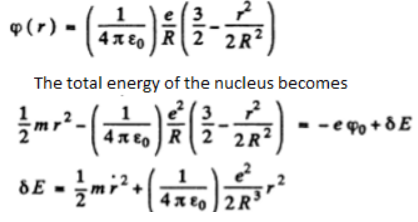This is the energy of a harmonic oscillator whose frequency is

$\omega =\frac{1}{4\pi \varepsilon _{o}}\frac{e^{2}}{mR^{3}}$

The vibrating electron emits radiation of frequency ω whose wavelength is

$\lambda =\frac{2\pi c}{\omega}=\frac{2\pi c}{e}\sqrt{mR^{3}}(4\pi \varepsilon _{o})^{\frac{1}{2}}$

In Gaussian units the factor $(4\pi \varepsilon _{o})^{\frac{1}{2}}$ is missing

Putting the values we get λ=0.237μm.

2. An alpha particle with kinetic energy 0.27 MeV is deflected through an angle of 60° by a golden foil. Find the corresponding value of the aiming parameter.

Solution:

1.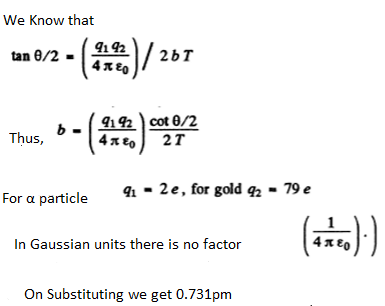3. To what minimum distance will an alpha particle with kinetic energy T = 0.40 MeV approach in the case of a head-on collision to

(a) a stationary Pb nucleus;

(b) a stationary free Liz nucleus?

Solution:

1. (a) In the Pb case, we shall ignore the recoil of the nucleus both because Pb is quite heavy(Apb= 208= 52 X AHe) as well as because Pb in not free. Then for a head-on collision, at the distance of closest approach, the K.E. of the α - particle must become zero (because α - particle will turn back at this point). Then

$\frac{2Ze^{2}}{(4\pi \varepsilon _{0})r_{min}}=T$

In Gaussian units the factor $(4\pi \varepsilon _{o})$ is missing. Om substituting values we get

rmin= 0.591pm

(b) Here we have to take account of the fact that part of the energy is spent in the recoil of Li nucleus. Suppose x1 coordinate of the α - particle from some arbitrary point on the line joining it to the Li nucleus, x2 = coordinate of the Li nucleus with respect to the same point Then we have the energy-momentum equations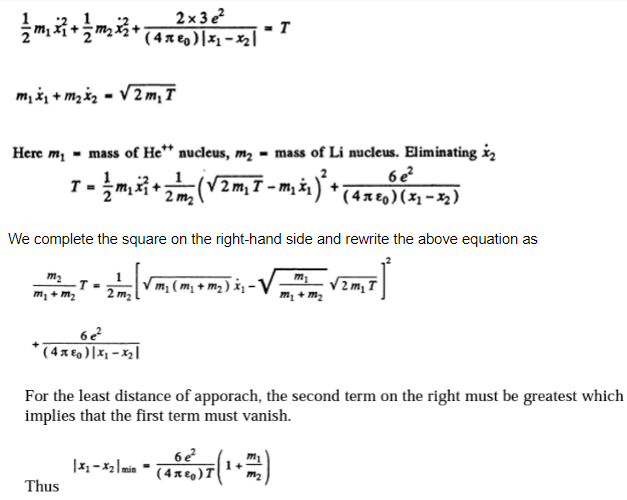Using m1/m2= 4/7 and substituting other values we get

|x1-x2|=034 pm.

In Gaussian units the factor $(4\pi \varepsilon _{o})$ is missing

4. An alpha particle with kinetic energy T = 0.50 MeV is deflected through an angle of θ = 90° by the Coulomb field of a stationary Hg nucleus. Find: All the formulas in this Part are given in the Gaussian system of units.

(a) the least curvature radius of its trajectory;

(b) the minimum approach distance between the particle and the nucleus

Solution:

1. We shall ignore the recoil of Hg nucleus.

(a) Let A be the point of closest approach to the centre C,AC=rmin

At A the motion is instantaneously circular because the radial velocity vanishes. Then if vo the particle at A, the following equations hold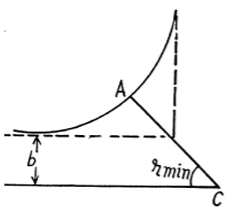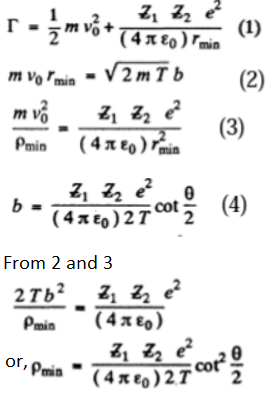With z1=2 and z2,=80 we get

ρmin= 0.231pm

*we used, δ=δmin is the radius of curvature of the path at A and P is minimum at A by symmetry and finally found equation (4)

(b) From equations 2 and 4 we get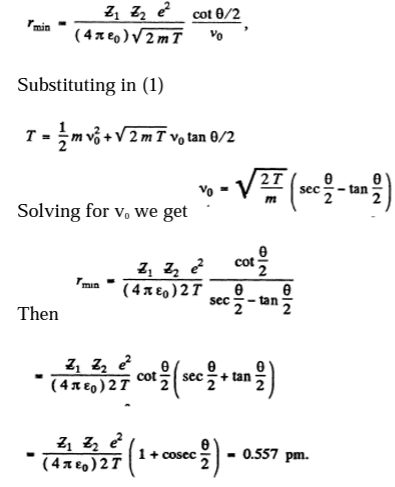5. A proton with kinetic energy T and aiming parameter b was deflected by the Coulomb field of a stationary Au nucleus. Find the momentum imparted to the given nucleus as a result of scattering.

Solution:

1. By momentum conservation

$\vec{P}+\vec{P_{i}}=\vec{P}+\vec{P_{f}}$

Thus the momentum transferred to the gold nucleus is clearly

$\vec{\Delta P}= \vec{P_{f}} -\vec{P_{i}}=\vec{P}-\vec{P}$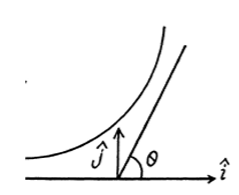Although the momentum transferred to the Au nucleus is not small, the energy associated with this recoil is quite small and its effect back on the motion of the proton can be neglected to a first approximation. Then

$\vec{\Delta P}= \sqrt{2mT}(1-\cos\theta )\hat{i}\sqrt{2mT}\sin\Theta \hat{j}$

Here $\hat{i}$ is the unit vector in the direction of the incident proton and $\hat{j}$ is normal to it on the side on which it is scattered. Thus,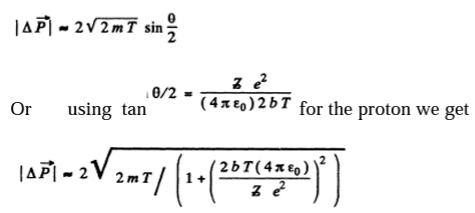6. A proton with kinetic energy T = 10 MeV flies past a stationary free electron at a distance b = 10 pm. Find the energy acquired by the electron, assuming the proton's trajectory to be rectilinear and the electron to be practically motionless as the proton flies by.

Solution:

1. The proton moving by the electron first accelerates and then decele rates and it is not easy to calculate the energy lost by the pro the componen t along OA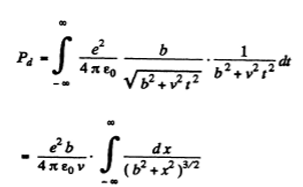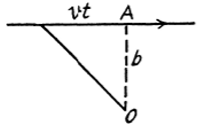Evaluate the integral by substituting

X-5 tan 8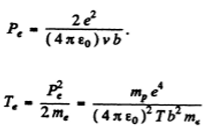In Gaussian units the factor $(4\pi \varepsilon _{o})^{2}$ is missing

Te= 3.82 eV

7. A particle with kinetic energy T is deflected by a radius R and depth Uo spherical potential well of,i.e. by the field in which the potential energy of the particle takes the form

$U=\left\{\begin{matrix}0 for, r>R & \\ & -U_{0} for, r

where r is the distance from the centre of the well. Find the relationship between the aiming parameter b of the particle and the angle 0 through which it deflects from the initial motion direction.

Solution:

1. In the region where potential is nonzero, the kinetic energy of the particle is, by energy conservation, T + U0 and the momentum of the particle has the magnitude $\sqrt{2m(T+U_{0})}$. On the boundary the force is radial, so the tangential component of the momentum does not change:

√(2mT) sinα = √2m(T-U0) sinϕ

So, sinϕ = √(T/T+U0) sinα = sinα/n

Where n = √(1+U0/T)

Θ = 2(α-ϕ)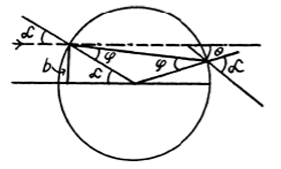$sin(\frac{\theta}{2})=sin(\alpha-\phi)= sin \alpha cos \phi-cos\alpha sin\phi$

= sinα(cos ϕ - cosα/n)

or $\frac{n sin(\theta/2)}{sin \alpha}=\sqrt{n^2-sin^2\alpha}-cos \alpha$

squaring both sides and solving, we have

cot α = [n cos(θ/2)-1]/nsin(θ/2)

Hence, sin α = [n sin(θ/2)]/√[1+n2-2n cos(θ/2)]

Finally, the impact parameter is

$b=R\sin\alpha =\frac{nR\sin\frac{\Theta }{2}}{\sqrt{1+n^{2}-2n\cos\frac{\Theta }{2}}}$

8. A stationary ball of radius R is irradiated by a parallel stream of particles whose radius is r. Assuming the collision of a particle and the ball to be elastic, find:

(a) the deflection angle θ of a particle as a function of its aiming parameter b;

(b) the fraction of particles which after a collision with the ball are scattered into the angular interval between θ and θ + dθ;

(c) the probability of a particle to be deflected, after a collision with the ball, into the front hemisphere. $\Theta < \frac{\pi }{2}$

Solution:

1. It is implied that the ball is too heavy to recoil.

(a) The trajectory of the particle is symmetrical about the radius vector through the point of impact. It is clear from the diagram that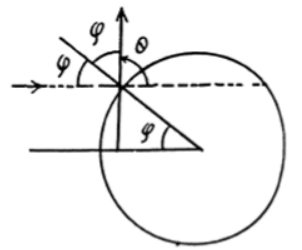$\Theta =\pi -2\phi$

Also $b=(R+r)\sin\phi = (R+r)\cos\frac{\Theta }{2}$

With b defined above, the fraction of the probability scattered between θ and θ + dθ or the probability of the same is

dP = |2πbdb|/π(R+r)2=(1/2) sinθ dθ

(c) $P =\int_0^{\pi/2}(1/2) sin\theta d\theta = (1/2)\int_{-1}^0d(-cos\theta) = 1/2$

9. A narrow beam of alpha particles with kinetic energy 1.0 MeV falls normally on a platinum foil 1.0 µm thick. The scattered particles are observed at an angle of 60° to the incident beam direction by means of a counter with a circular inlet area of 1.0 cm2 located at a distance of 10 cm from the scattering section of the foil. What fraction of scattered alpha particles reaches the counter inlet?

Solution:

1. We know that

$\frac{dN}{N}=n(\frac{ze^2}{4 \pi \epsilon_o)2T})^2 \frac{dQ}{sin^4(\theta/2)}$

on substituting q1 =2e, q2=ze

Here n= number of platinum nuclei in the foil per unit area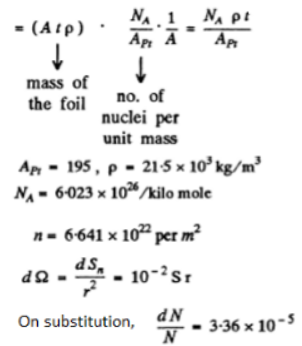10. A narrow beam of alpha particles with kinetic energy T = = 0.50 MeV and intensity I = 5.0.105particles per second falls normally on a golden foil. Find the thickness of the foil if at a distance r = 15 cm from a scattering section of that foil the flux density of scattered particles at the angle θ = 60° to the incident beam is equal to J = 40 particles/(cm2•s).

Solution:

1. A scattered flux density of J (particles per unit area per second) equals $\frac{J}{\frac{1}{r^{2}}}=r^{2}J$ particles scattered per unit time per steradian in the given direction. Let n = concentration of the gold nuclei in the foil. Then

$n=\frac{N_{a}\rho }{A_{Au}}$

and the number of Au nuclei per unit area of the foil is nd, where d = thickness of the foil

Then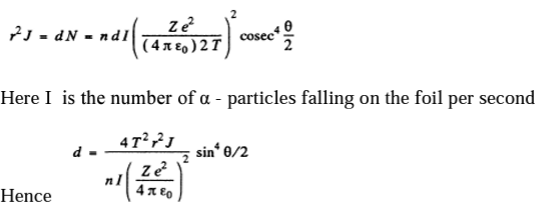Using Z=79, Aau= 197, ρ= 193X 103kg/m3, NA= 6.023 X 1026 kilo mole and other data from the problem we get

d=1.47pm

11. A narrow beam of alpha particles falls normally on a silver foil behind which a counter is set to register the scattered particles. On substitution of platinum foil of the same mass thickness for the silver foil, the number of alpha particles registered per unit time increased η = 1.52 times. Find the atomic number of platinum, assuming the atomic number of silver and the atomic masses of both platinum and silver to be known.

Solution:

1. We know that

dNPt/dNAg = nPt/nAg . = η

The foils have same mass thickness=pd, we get

nPt/nAg = AAg/APt

Hence, ZPt = ZAg √[ηAAg/APt]

Put ZAg = 47, AAg = 108

12. A narrow beam of alpha particles with kinetic energy T = = 0.50 MeV falls normally on a golden foil whose mass thickness is pd = 1.5 mg/cm2. The beam intensity is I0 = 5.0.105 particles per second. Find the number of alpha particles scattered by the foil during a time interval ζ = 30 min into the angular interval:

(a) 59-61°

(b) over θ0 = 60°

Solution:

1. We know that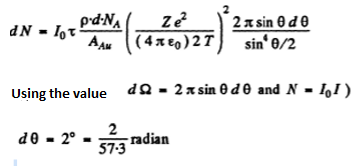Also Z Au = 79 , AAm=197

On substituting these values we get

dN = 1.63 x 106

(b) The number is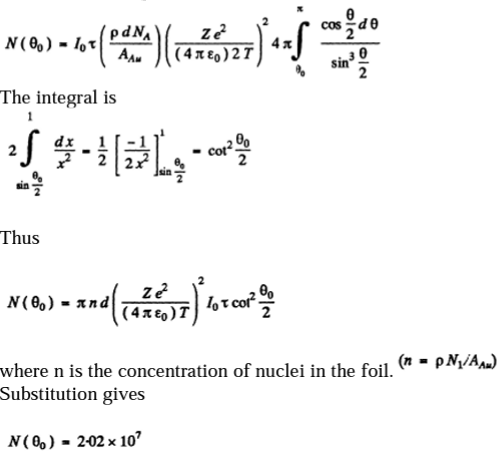13. A narrow beam of protons with velocity v = 6.106m/s falls normally on a silver foil of thickness d = 1.0 p,m. Find the probability of the protons being scattered into the rear hemisphere (θ > 90°).

Solution:

1. The requisite probability can be written easily by analogy with (b) of the previous problem. It is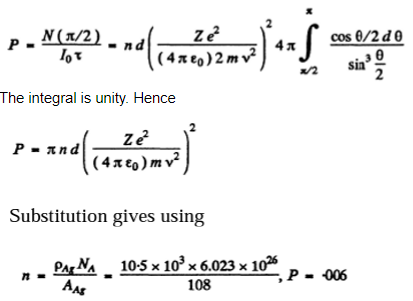14. A narrow beam of alpha particles with kinetic energy T = = 600 keV falls normally on a golden foil incorporating n = 1.1.1019 nuclei/cm2. Find the fraction of alpha particles scattered through the angles θ < θ0 = 20°.

Solution:

1. Because of the $\csc ^{4}\frac{\Theta }{2}$dependence of the scattering, the number of particles (or fraction) scattered through θ < θO cannot be calculated directly. But we can write this fraction as

$P(\Theta _{0})=1-Q(\Theta _{0})$

where Q (θO) is the fraction of particles scattered through $\Theta\geq Q(\Theta _{0})$

This fraction has been calculated before and is

$Q(\Theta _{0})=\pi n\left [ \frac{Ze^{2}}{(4\pi \varepsilon _{0})T})^{2}\right ]\cot^{2}\frac{\Theta _{0}}{2}$

where n here is number of nuclei/cm2 .

Using the data we get Q = 0.4

$P(\Theta _{o})=6$

15. A narrow beam of protons with kinetic energy T = 1.4 MeV falls normally on a brass foil whose mass thickness pd = 1.5 mg/cm2. The weight ratio of copper and zinc in the foil is equal to 7 : 3 respectively. Find the fraction of the protons scattered through the angles exceeding θ0= 30°

Solution:

1. We know that

The relevant fraction can be immediately written down

$\frac{\Delta N}{N}=\left [ \frac{e^{2}}{(4\pi \varepsilon _{0})2T} \right ]^{2}\pi \cot^{2}\frac{\theta _{o}}{2}.(n_{1}.Z_{1}^{2}n_{2}.Z_{2}^{2})$

Here n1 (n2) is the number of Zn(Cu) nuclei per cm2 of the foil and Z1(Z2) is the atomic number of Zn(Cu). Now

$n_{1}=\frac{\rho dN_{A}}{M_{1}}=0.7$

$n_{2}=\frac{\rho dN_{A}}{M_{2}}=0.3$

Here M1 , M 2 are the mass numbers of Zn and Cu. Then, substituting the values Z1 = 30, Z2 = 29, M1 = 65.4, M2 = 63.5, we get

$\frac{\Delta N}{N}=1.43\times10^{-3}$

16. Find the effective cross-section of a uranium nucleus corresponding to the scattering of alpha particles with kinetic energy T = 1.5 MeV through the angles exceeding θ0 = 60°

Solution:

1. From Rutherford scattering formula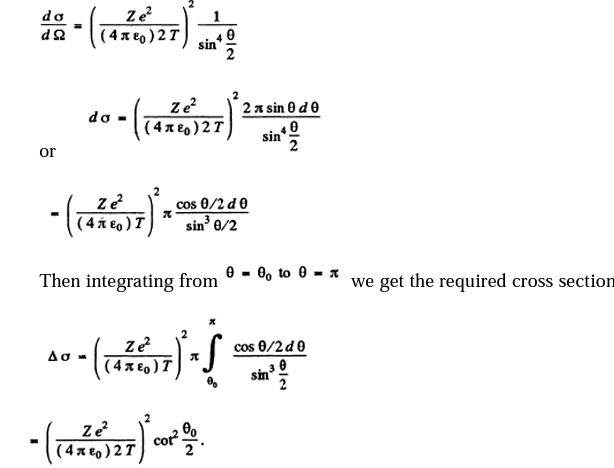For Uranium nucleus Z - 92 and on substituting the values we get

$\Delta \sigma =737b=0.737kb$

17. The effective cross-section of a gold nucleus corresponding to the scattering of monoenergetic alpha particles within the angular interval from 90° to 180° is equal to Δσ = 0.50 kb. Find:

(a) the energy of alpha particles;

(b) the differential cross-section of scattering dσ/dΩ (kb/sr) corresponding to the angle θ = 60°.

Solution:

1. (a)From the previous formula, we know that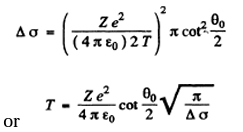Substituting the values with Z = 79

we get (θO = 90°)

T = 0.903 MeV

(b) The differential scattering cross section is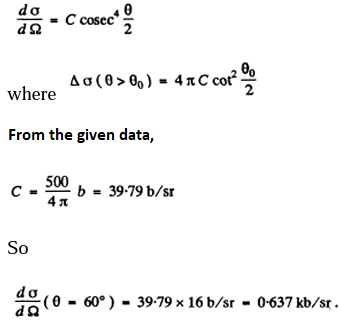18. In accordance with classical electrodynamics, an electron moving with acceleration ω loses its energy due to radiation as

$\frac{dE}{dT}=\frac{-2e^{2}}{-3e^{2}}w^{2}$

where e is the electron charge, c is the velocity of light. Estimate the time during which the energy of an electron performing almost harmonic oscillations with frequency ω = 5.1015 s-1 will decrease η = 10 times.

Solution:

1. The formula in MKS units is

$\frac{dE}{dT}=\frac{\mu _{0}e^{2}}{6\pi c}\vec{w^{2}}$

For an electron performing (linear) harmonic vibrations $\vec{w}$ is in some definite directions with

Assume wx=w2x

Thus,

$\frac{dE}{dT}=\frac{\mu _{0}e^{2}\omega ^{4}}{6\pi c}{x^{2}}$

If the radiation loss is small (i.e. if ω is not too large), then the motion of the electron is always close to simple harmonic with slowly decreasing amplitude. Then we can write

$E=\frac{1}{2}m\omega ^{2}a^{2}$

$x=a\cos\omega t$

and average the above equation ignoring the variation of a in any cycle. Thus we get the equation, on using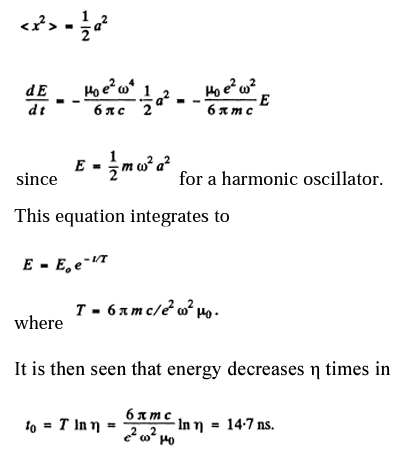19. Making use of the formula of the foregoing problem, estimate the time during which an electron moving in a hydrogen atom along a circular orbit of radius r = 50 pm would have fallen onto the nucleus. For the sake of simplicity assume the vector ω to be permanently directed toward the centre of the atom.

Solution:

1. Moving around the nucleus, the electron radiates and its energy decreases. This means that the electron gets nearer the nucleus. By the statement of the problem, we can assume that the electron is always moving in a circular orbit and the radial acceleration by Newton’s law is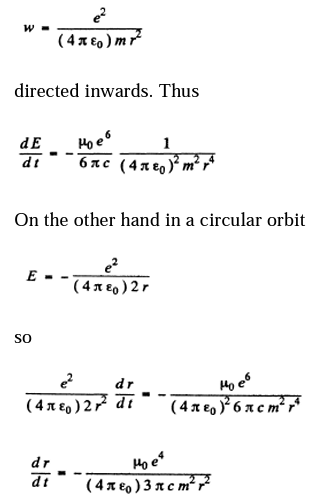On integrating the above equation we get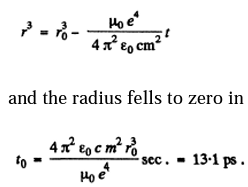20. Demonstrate that the frequency ω of a photon emerging when an electron jumps between neighbouring circular orbits of a hydrogen-like ion satisfies the inequality ωn > ω > ωn +1, where ωn and ωn +1 are the frequencies of revolution of that electron around the nucleus along the circular orbits. Make sure that as n → ∞ the frequency of the photon ω → ωn.

Solution:

1. In a circular orbit we have the following formula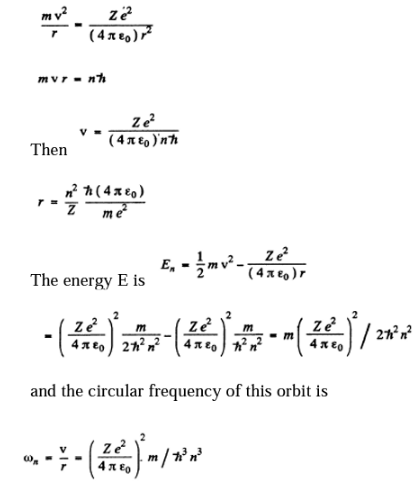On the other hand the frequency of the light emitted when the electron makes a transition n + 1 → n is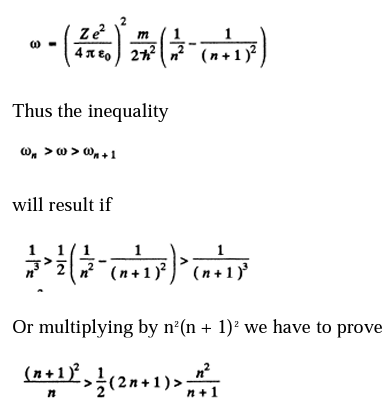The above equation can also be written as